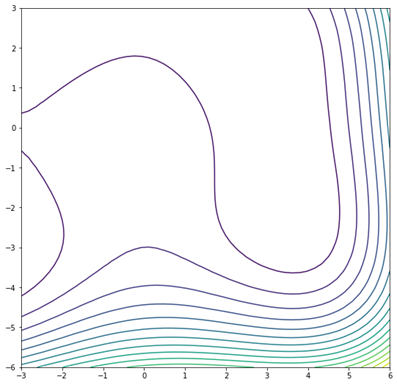top of page
Search

# HIMMELBLAU FUNCTIONMathematical DefinitionInput Domain

The function is defined on input range xi ∈[−6, 6] for i=1, 2.

Global Minima

The Himmelblau Function has four identical local minimum at:

• f(x∗)=0 at x∗=(3, 2)

• f(x∗)=0 at x∗=(−2.805118, 3.283186)

• f(x∗)=0 at x∗=(−3.779310, −3.283186)

• f(x∗)=0 at x∗=(3.584458, −1.848126)

The function has one local maximum at x=-0.270845 and y=-0.923039 where f(x, y)=181.617.

Description and Features

This function is named after David Mautner Himmelblau who introduced it. The Himmelblau Function is defined on the two dimensional space, used to test the performance of optimization algorithms such as:

• Convergence rate

• Precision

• Robustness

The Himmelblau function is a

• Multi-modal function

• Non-separable

• Non-convex

• Continuous

Python Implementation

```% Please forward any comments or bug reports in chat
Copyright 2021. INDUSMIC PRIVATE LIMITED.THERE IS NO WARRANTY, EXPRESS OR IMPLIED. WE DO NOT ASSUME ANY LIABILITY FOR THE USE OF THIS PROGRAM. If software is modified to produce derivative works, such modified software should be clearly marked. Additionally, user can redistribute it and/or modify it under the terms of the GNU General Public License. This program is distributed in the hope that it will be useful, but WITHOUT ANY WARRANTY. See the GNU General Public License for more details.
% for any support connect with us on help.indusmic@gmail.com
% Author: RIYA SHIVHARE

import matplotlib.pyplot as plt
import numpy as np
from mpl_toolkits import mplot3d

def f(x,y):
return (((x**2+y-11)**2) + (((x+y**2-7)**2)))

X=np.linspace(-6,6)
Y=np.linspace(-6,6)

x,y=np.meshgrid(X,Y)
F=f(x,y)

fig =plt.figure(figsize=(9,9))
ax=plt.axes(projection='3d')
ax.contour3D(x,y,F,450)

ax.set_xlabel('X')
ax.set_ylabel('Y')
ax.set_zlabel('F')
ax.set_title('Himmelblau Function')
ax.view_init(50,50)

#plt.contour(x,y,F,15)
plt.show()

```

References:

 Jamil, Momin, and Xin-She Yang. "A literature survey of benchmark functions for global optimization problems." International Journal of Mathematical Modelling and Numerical Optimization 4.2 (2013): 150-194.